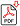Search for Articles:

## Arithmetic Gates as Part of Mathematical Gates Theory and Physics Gates Theory

Engr. Dr. Inv. Ismael Tabu?ar Fortunado

Abstract: There are many logic gates. There is arithmetic and logic unit, an essential part of computers. Computers and circuits use logic and mathematics. 1 and 0 represents true and false, on and off, mathematical values. There are four arithmetic operations namely addition, subtraction, multiplication and division. This paper is a representation and part operation of proposed arithmetic gates and an introduction to mathematical gates theory.

Keywords: Arithmetic and Logic Unit Calculus Logic Logic Gates Mathematics

Country: Philippines, Subject Area: Biochemistry

Pages: 203 - 203

Edition: Volume 8 Issue 7, July 2019